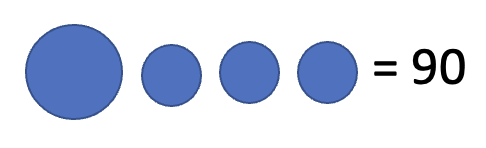top of pageSearch

# Math Competition Problems - Problem of the Week - 20

Updated: Jun 17, 2021

Andrew is doing some tidying. He can tidy 2 big rooms in the same time it takes to tidy 3 small rooms. He can tidy one big room and three small rooms in 90 minutes. How long will it take him to tidy 3 big rooms and 6 small rooms? - AMC (Australian Mathematical Competition) Upper Primary 2018, Question 20Let a big circle represent the time taken to tidy a big room.

Let a small circle represent the time taken to tidy a small room

Since he can tidy 2 big rooms at the same time as 3 small rooms,

2 big circles = 3 small circlesHe can tidy 1 big room and 3 small rooms in 90 mins.

So, 1 big circle + 3 small circles = 90 minsWe can replace the 3 small circles with 2 big circles because they are equivalent.

Hence our equation becomes 3 big circles = 90 minsHence one big circle has to be 30 mins. Which means he takes 30 mins to clean the big roomSince,We can replace the big circle with 30 and get

30 + 3 small circles = 90So we know the 3 small circles have to be 60.Which means 1 small circle = 20. So he takes 20 minutes to tidy a small room.So Andrew takes 30 minutes to tidy 1 Big Room

and 20 minutes to tidy 1 Small Room.

We know it is right because time taken for 2 big rooms is 30*2=60 and time taken for 3 small rooms is 20*3=60 and they are equal.

Which means for 3 big rooms it will take him 90 minutes and 6 small rooms it will take him 120 minutes, giving a total of 210 minutes or 3 hours and 30 minutes.

Want to prepare for AMC? Working out the problems by yourself is the only way to prepare for it.

### Try out a similar problem.

5 Oranges cost the same as 4 Apples. If 4 Apples and 4 Oranges cost \$5.40, then how much does 5 Oranges and 3 Apples cost?Interesting Math Problem 113

Did you like the problem? Would you like to have these problems and solutions delivered to your inbox as I publish them? Click here to subscribe

Previous Week's Problem

Problem of the Week 19

Next Week's Problem